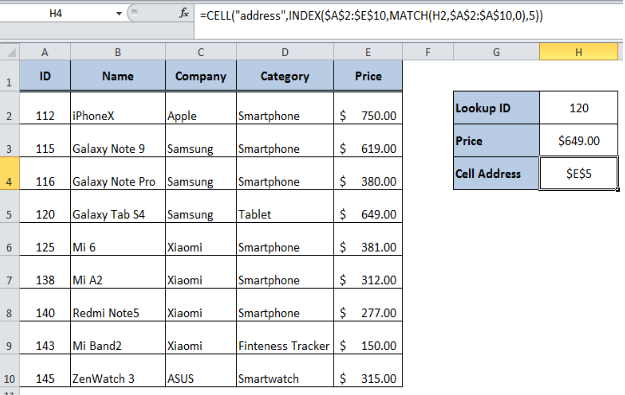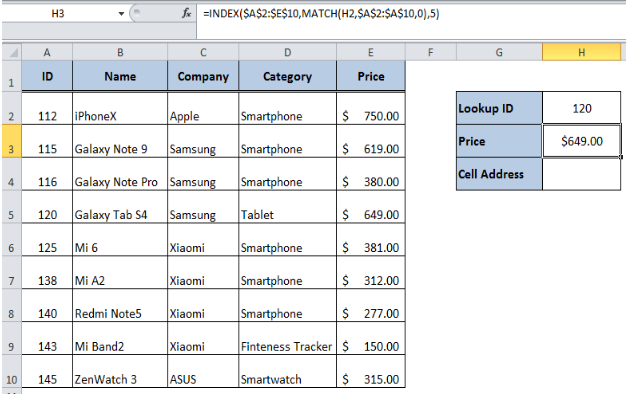Get instant live expert help with Excel or Google Sheets“My Excelchat expert helped me in less than 20 minutes, saving me what would have been 5 hours of work!”

#### Post your problem and you’ll get Expert help in seconds.

Your message must be at least 40 characters
Our professional Expert are available now. Your privacy is guaranteed.

# How to Get Address of Lookup Result

Generally, we look up for the required information in a large dataset using INDEX, MATCH functions and return the matching value based on given criteria. But if we want to look up a value and return the cell reference or the cell address of resulting value then we need to use the CELL function. In this article, we will learn how to lookup for a value and get the address of lookup result using the CELL function.Figure 1. Final result

## CELL Function

The CELL function gives information about a specific cell in a worksheet. That information can either be things like address and filename or even much more detailed, such as cell formatting, etc.

CELL Syntax:

`=CELL(“address”,[reference])`

This formula syntax uses following arguments to get the address of lookup result:

• address (required): This is the type of information that you are returning about the reference.
• reference (optional): This is a reference or range of cells to return the lookup result

## Lookup Result

As per the formula syntax, the CELL function requires the cell reference of lookup result and normally the INDEX function returns the cell values of the lookup result. However, the INDEX function returns the cell reference of the lookup result. Therefore, we need to use the INDEX and MATCH functions for the lookup results and then wrap them inside the CELL function as a reference argument to get the address of the lookup result. For example, we have a data set of various products in inventory and we need a lookup result of price for a lookup ID 120 in cell H3 using the following INDEX, MATCH formula:

`=INDEX(\$A\$2:\$E\$10,MATCH(H2,\$A\$2:\$A\$10,0),5)`Figure 2. Lookup Result of Price of an item

## Get Address of Lookup Result

Now, we want to get the address of lookup result of price as mentioned in cell H3. In order to do that we will wrap the INDEX, MATCH formula in CELL function using “address” option in cell H4 in the following formula.

`=CELL("address",INDEX(\$A\$2:\$E\$10,MATCH(H2,\$A\$2:\$A\$10,0),5))`

The INDEX function returns the reference of lookup result of price in range A2: E10 based on row and column number. The MATCH function returns the relative position (row number) for lookup ID 120 in range A2: A10. Since the price is listed in the 5th column of range A2: E10, we need to mention the column_num argument as 5. When we wrap the INDEX, MATCH functions inside the CELL function with “address” option it returns the cell address of lookup result of price in cell H4. It means that lookup result is present in \$E\$5 as per this example.Figure 3. Cell address of lookup result of Price

## Instant Connection to an Expert through our Excelchat Service:

Most of the time, the problem you will need to solve will be more complex than a simple application of a formula or function. If you want to save hours of research and frustration, try our live Excelchat service! Our Excel Experts are available 24/7 to answer any Excel question you may have. We guarantee a connection within 30 seconds and a customized solution within 20 minutes.

### Did this post not answer your question? Get a solution from connecting with the expert.Another blog reader asked this question today on Excelchat:
Solution examplesindex and match with duplicates. I need to use another column as a reference, so my return value has two match the value of two things for it to return
Solved by E. H. in 60 minsHello, I have a big spreadsheet in which I need to know how many patients came in each month based on provider. I am using the following formula but I still get the N/A error. =INDEX('No Show Appts Data'!D:E, MATCH(1, 'No Show Appts Data'!D:D='No Shows Data'!M2)*('No Shows Data'!E:E='No Shows Data'!N1),0)) No Show Appts Data is the name of the sheet where Column D is Month Year of date in question and Column E is the provider. No Shows Data is the sheet where I am making all the formula calculation where Column M is Month and Year and Column N is the provider in question and therefore M2 is the month in question and N1 the provider in question. How do I fix this error? Thanks
Solved by F. H. in 40 minsI need to find an INDEX function that will convert the Call Day (a number 1-7) to the actual weekday found in row 1 of the DayofWeek named range. I don't know if I'm supposed to use the MATCH function as well or not.
Solved by D. D. in 11 minsI have two columns with names and a third one with email addresses. For every name that matches, I need to copy the email address to an empty column next to the matching name. .
Solved by E. U. in 15 minshelp with INDEX(IndexArea,MATCH(\$M\$5,MatchRows,0),MATCH(\$M\$6,MatchColumns,0))
Solved by Z. Y. in 12 mins## Subscribe to Excelchat.coAnother blog reader asked this question today on Excelchat: